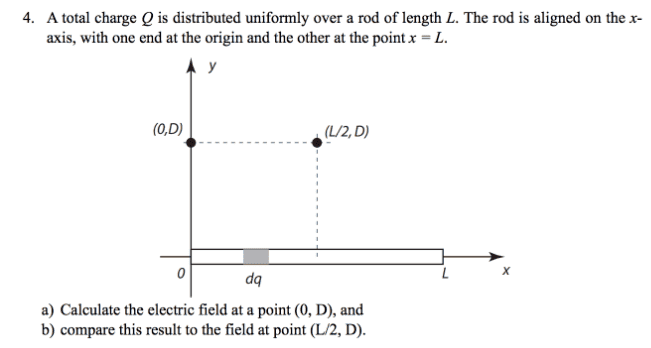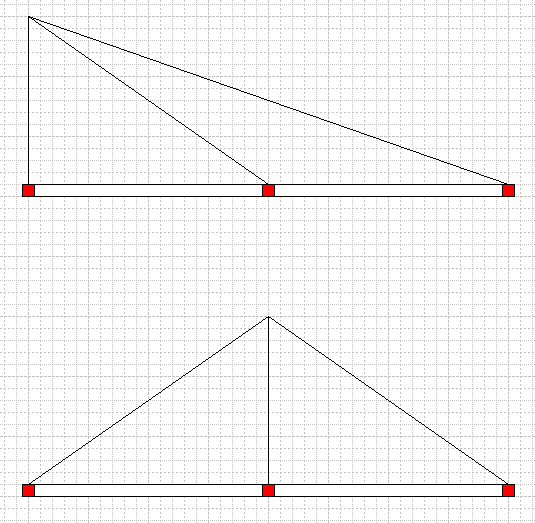# Electric field due to a charged rod

## Homework Statement[moderator edit: Image inserted for clarity]
A total charge Q is distributed uniformly over rod length L. The rod is aligned on the x-axis, with one end at the origin and the other at point x=L.
a) calculate electric field at a point (0,D)
b) field at point (L/2,D)

## Homework Equations

E=Kq/r^2
q = lambda*length

## The Attempt at a Solution

I was able to find the answer to letter a. However, I deduce that for b), the answer will only be the y-component of that found in a) as the x one cancel out. However, this is not the case as the answer key shows.

Thus, I tried doing the calculations and I still arrive at the y-component found in a).

Thanks

Last edited by a moderator:

gneill
Mentor
Hi Jabbar, Welcome to Physics Forums!

•Jabbar
andrevdh
Homework Helper
Part b is very much like part a if you consider sliding the rod halfway to the left

SammyS
Staff Emeritus
Homework Helper
Gold Member

## Homework Statement

View attachment 106377
[moderator edit: Image inserted for clarity]
A total charge Q is distributed uniformly over rod length L. The rod is aligned on the x-axis, with one end at the origin and the other at point x=L.
a) calculate electric field at a point (0,D)
b) field at point (L/2,D)

## Homework Equations

E=Kq/r^2
q = lambda*length

## The Attempt at a Solution

I was able to find the answer to letter a. However, I deduce that for b), the answer will only be the y-component of that found in a) as the x one cancel out. However, this is not the case as the answer key shows.

Thus, I tried doing the calculations and I still arrive at the y-component found in a).

Thanks
How do your results for the x component compare in parts (a) and (b) ?

•Jabbar

## Homework Statement

https://gyazo.com/73616d3f59649b888b3aa465ec850bf7 (Correct Answers to problem: #4).
View attachment 106377
[moderator edit: Image inserted for clarity]
A total charge Q is distributed uniformly over rod length L. The rod is aligned on the x-axis, with one end at the origin and the other at point x=L.
a) calculate electric field at a point (0,D)
b) field at point (L/2,D)

## Homework Equations

E=Kq/r^2
q = lambda*length

## The Attempt at a Solution

I was able to find the answer to letter a. However, I deduce that for b), the answer will only be the y-component of that found in a) as the x one cancel out. However, this is not the case as the answer key shows. I then also calculated it manually and still got the y-component found in a)

b)

Thus, I tried doing the calculations and I still arrive at the y-component found in a) :

dE=k*dq/r^2

dE=k*dq/r^2

dE=k*dq/r^2

dE=k*dq/(x^2+D^2)

dEy=k*dq/(x^2+D^2) *sin theta

dEy=(k*dx*lambda)/((x^2+D^2)^3/2)

taking the integral from 0 to L I get: Ey= k*lambda*D* [x/D^2*sqrt(x^2+D^2)]

Ey= k*lambda*L/D*sqrt(x^2+D^2)

Thanks
Hi Jabbar, Welcome to Physics Forums!

gneill
Mentor
Can you explain what you used to substitute for sin(θ)? I'd expect to see a 'D' in the numerator as a result of the substitution.

Since the x-components cancel by symmetry, if you slide the rod to the left so that its center point is at the origin and half the rod is to the left of the origin and half to the right (this was suggested previously by @andrevdh) then you can take further advantage of symmetry and integrate from x = 0 to x = L/2. Just multiply the result by two.

Does the answer key solution keep the charge density as λ or does it insert Q/L for it?

•Jabbar
This is my theta.

For the second part, yeah I see that if I do that I can integrate quite easily from either -L/2 to L/2 or "x = 0 to x = L/2. Just multiply the result by two". Thanks for the insight.

I just don't know why what I'm doing is wrong. Moreover, why can't I say that its electric field will be equal to the y-component found in a). It feels logical to me. I'd really like to understand the concept :( .

Thanks

#### Attachments

gneill
Mentor
I just don't know why what I'm doing is wrong. Moreover, why can't I say that its electric field will be equal to the y-component found in a). It feels logical to me. I'd really like to understand the concept :( .
Consider that the field strength due to some dq on the rod varies inversely with the square of the distance between the point of interest and the dq. Then consider the average distance of the dq's in each case. Which case should have a larger sum of contributions?

Consider that the field strength due to some dq on the rod varies inversely with the square of the distance between the point of interest and the dq. Then consider the average distance of the dq's in each case. Which case should have a larger sum of contributions?

It's going to stay the same no? As the distance the two points have to dq are the same.

gneill
Mentor
It's going to stay the same no? As the distance the two points have to dq are the same.
Consider the two cases:The average line distances up to the midpoint in the first case are the same as those on both sides of the point of interest in the second case. After the midpoint in the first case every distance is longer than any distance in the second case.

Consider the two cases:
View attachment 106442
The average line distances up to the midpoint in the first case are the same as those on both sides of the point of interest in the second case. After the midpoint in the first case every distance is longer than any distance in the second case.
Oh alright, so the Electric field at that point in a) will be smaller than in b) since for dqs' on the rod... the first case will have dqs' with a greater distance r. right?

gneill
Mentor
Oh alright, so the Electric field at that point in a) will be smaller than in b) since for dqs' on the rod... the first case will have dqs' with a greater distance r. right?
Right. More of the charge is further away.

•Jabbar
Right. More of the charge is further away.
Thanks you very much!!!! and just for my calculation mistake. Is it just that it is better to have the point of interest along one axis to do a problem. Because won't changing the axis affect my results? I can't possible change my axis mid problem no?

Thanks

gneill
Mentor
Thanks you very much!!!! and just for my calculation mistake. Is it just that it is better to have the point of interest along one axis to do a problem. Because won't changing the axis affect my results? I can't possible change my axis mid problem no?

Thanks
In this problem they're asking for the electric field which is a vector quantity: As long as you don't rotate the axes it won't change the result.

•Jabbar